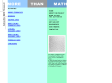Public marks with tags math:geometry & gr4 http://blogmarks.net/marks/tag/math:geometry,gr4 More Than Math http://www.morethanmath.org/activities.htm More Than Math integrates the visual arts into the 3rd, 4th and 5th grade mathematics curriculum using works of art to explore pattern, symmetry, proportion, perspective, balance and geometric form 2005-09-26T16:59:45Z cherbourg gr3, gr4, gr5, sesvt, ssdvt, math skills, math:geometry, math toolMore Than Mathby cherbourg & 1 other (via)
More Than Math integrates the visual arts into the 3rd, 4th and 5th grade mathematics curriculum using works of art to explore pattern, symmetry, proportion, perspective, balance and geometric form
]]>